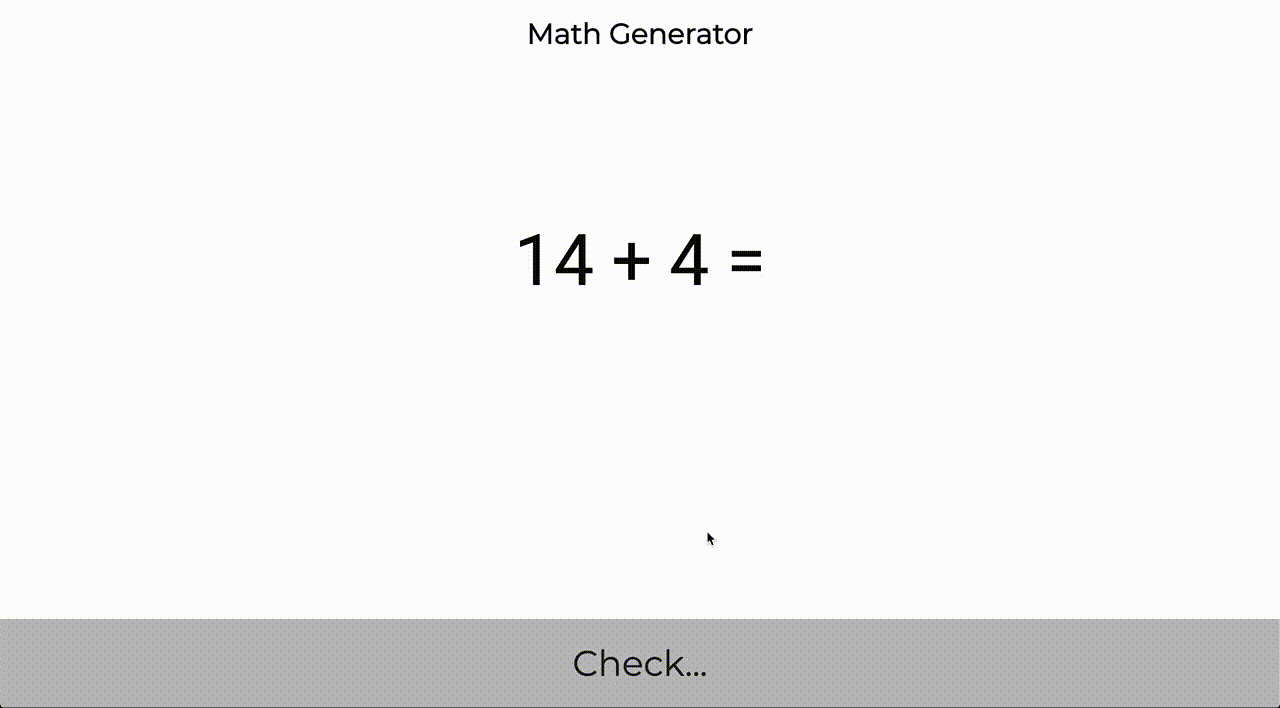Version: v0.1.0 Latest LatestGo to latest
Published: Jan 26, 2020 License: MIT

##README ¶

### Math generatorAs a dad, I frequently to play math quiz with my daughter. Bu I'm too lazy to frequently to create math questions. This' reason why I create this lib/api/web, that I can help me to generate questions. This repository will evolue frequently, I think, to support more topic.

#### Use as library

Install library:

``````  go get -u github.com/ledongthuc/mathgen
``````

Usage:

``````  questionAndAnswers, _ := mathgen.AddIntegerN(3, 20)
``````

More detail supported functions is cover at: https://godoc.org/github.com/ledongthuc/mathgen

#### Run demo application

##### Startup

``````docker run -p 8080:8080 ledongthuc/mathgen-web:latest
``````

Start from source code:

``````make clean; make test; make run;
``````
##### API

POST `http://localhost:8080/api/addition/generate`

Request:

``````{
"max_sum": 10
}
``````

Response:

``````{
3,
4
],
"sum": 7,
"question": "3 + 4 = ",
"result": "3 + 4 = 7"
}
``````
###### Subtraction

POST `http://localhost:8080/api/subtraction/generate`

Request:

``````{
"max_minuend": 20,
"number_of_subtrahends": 1
}
``````

Response:

``````{
"minuend": 9,
"subtrahends": [
5
],
"difference": 4,
"question": "9 - 5 = ",
"result": "9 - 5 = 4"
}
``````
##### Web

Local: http://localhost:8080

Free site: https://mathgen.thuc.space##Documentation ¶

### Overview ¶

Package mathgen is a library for math's questions & answers generation.

### Constants ¶

This section is empty.

### Variables ¶

This section is empty.

### Functions ¶

This section is empty.

### Types ¶

```type AdditionResult struct {
Sum     int64
}```

`func AddIntegerN(numberOfAddends int, maxSum int64) (AdditionResult, error)`

AddIntegerN generate a operator with sum. Parameter numberOfAddends defines addends in question, it should be greater than 1. Parameter maxSum defines maxsimum of Sum result, it should be greater than 0. If maxSum is greater than or equal numberOfAddends. Question always has pattern: 1 + 1 + 1 ....

Example
```package main

import (
"fmt"

"github.com/ledongthuc/mathgen"
)

func main() {
}
```
```Output:

```

`func AddIntegers(maxSum int64) (AdditionResult, error)`

AddIntegers generate a operator with sum of 2 addends. Parameter maxSum defines maxsimum of Sum result, it should be greater than 0. If maxSum = 2, addends always is 1 + 1

Example
```package main

import (
"fmt"

"github.com/ledongthuc/mathgen"
)

func main() {
}
```
```Output:

```

`func (a AdditionResult) String() string`

String of AdditionResult will present struct data with question answer format a + b + c + ... = sum

`func (a AdditionResult) StringQuestion() string`

StringQuestion of AdditionResult will present struct data with question format a + b + c + ... =

#### type SubtractionResult ¶

```type SubtractionResult struct {
Minuend     int64
Subtrahends []int64
Difference  int64
}```

#### func SubtractIntegerN ¶

`func SubtractIntegerN(numberOfSubtrahends int, maxMinuend int64) (SubtractionResult, error)`

SubtractIntegerN generate a operator with subtraction. Parameter numberOfSubtrahends defines subtrahends in question, it should be greater than 0. Parameter maxMinuend defines maxsimum of minuend result, it should be greater than 0. If maxMinuend = 1, addends always is 1 - 1 - 0 ....

Example
```package main

import (
"fmt"

"github.com/ledongthuc/mathgen"
)

func main() {
}
```
```Output:

```

#### func SubtractIntegers ¶

`func SubtractIntegers(maxMinuend int64) (SubtractionResult, error)`

SubtractIntegers generate a operator with subtraction of 1 minuend and 1 subtrahend. Parameter maxMinuend defines maxsimum of minuend, it should be greater than 0. If maxMinuend = 1, addends always is 1 - 1

Example
```package main

import (
"fmt"

"github.com/ledongthuc/mathgen"
)

func main() {
}
```
```Output:

```

#### func (SubtractionResult) String ¶

`func (s SubtractionResult) String() string`

String of SubtractionResult will present struct data with question answer format a - b - c - d ... = difference

#### func (SubtractionResult) StringQuestion ¶

`func (s SubtractionResult) StringQuestion() string`

StringQuestion of SubtractionResult will present struct data with question format a - b - c - d ... =

Path Synopsis